## FISHEYE2PANO

 Generates a strip panoramic image from a linear type fisheye image with optional vertical perspective correction.

### EXAMPLES

 Linear Circular Fisheye Image Panoramic Vertical View Range: -90 deg to 0 deg = 90 deg Fisheye Field Of View: 2*90 = 180 deg Nadir At Center http://local.wasp.uwa.edu.au/~pbourke/projection/fish2/ OriginalDiagram Of LookDirections For PanoramasExample 1: Format: Angle Horizontal Center = 0 Deg Arguments: -v -90,0 -h 0 -f angle Diagram Rotate So That Center DirectionIs At Top For Easier ComparisonExample 2: Format: Angle Horizontal Center = 90 Deg Arguments: -v -90,0 -h 90 -f angle Diagram Rotate So That Center DirectionIs At Top For Easier Comparison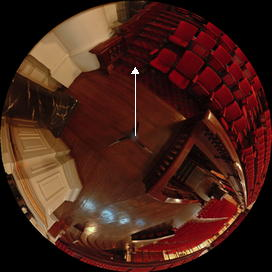Example 3: Format: Angle Horizontal Center = 180 Deg Arguments: -v -90,0 -h 180 -f angle Diagram Rotate So That Center DirectionIs At Top For Easier Comparison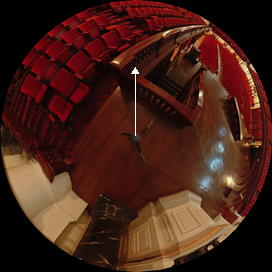Example 4: Format: Angle Horizontal Center = 270 Deg Arguments: -v -90,0 -h 270 -f angle Diagram Rotate So That Center DirectionIs At Top For Easier Comparison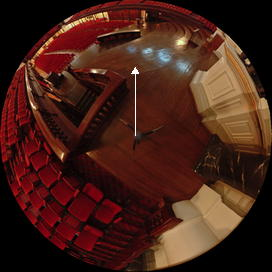Example 5: Format: Tilt (Perspective) Horizontal Center = 0 Deg Arguments: -v -90,0 -h 0 -f tilt Diagram Rotate So That Center DirectionIs At Top For Easier Comparison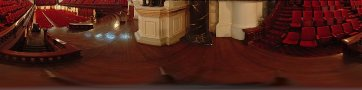Example 6: Format: Angle Horizontal Center = 0 Deg Width = 500 Arguments: -v -90,0 -h 0 -f angle -w 500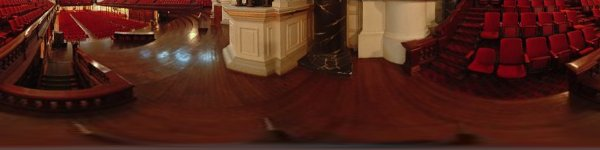Example 7: Format: Tilt (Perspective) Horizontal Center = 0 Deg Width = 500 Arguments: -v -90,0 -h 0 -f tilt -w 500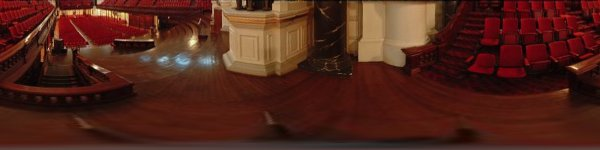Hyperwide (Panoramic) Fisheye Image Panoramic Vertical View Range: -62.5 deg to 52.5 deg = 115 deg Fisheye Field Of View: 2*(52.5 - (-90)) = 285 deg Nadir At Center http://www.0-360.com/index.asp OriginalDiagram Of LookDirection For PanoramaExample 1: Format: Angle Horizontal Center = 211.5 Deg Arguments: -v -90,52.5 -h 211.5 -f angle Diagram Rotate So That Center DirectionIs At Top For Easier ComparisonExample 2: Format: Tilt Horizontal Center = 211.5 Deg Persp. FOV = ifov/2 = 142.5 Deg Arguments: -v -90,52.5 -h 211.5 -f tilt Diagram Rotate So That Center DirectionIs At Top For Easier ComparisonHyperwide (Panoramic) Fisheye Image Panoramic Vertical View Range: -20 deg to 90 deg = 110 deg Fisheye Field Of View: 2*(90 - (-20)) = 220 deg Zenith At Center Caesar's Palace, Las Vegas OriginalDiagram Of LookDirections For PerspectivesExample 1: Format: Angle Horizontal Center = 0 Deg Arguments: -v 90,-20 -h 0 -f angle Diagram Rotate So That Center DirectionIs At Bottom For Easier Comparison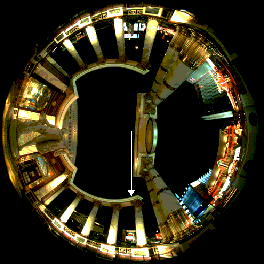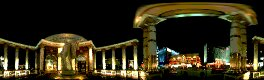Example 2: Format: Angle Horizontal Center = 90 Deg Arguments: -v 90,-20 -h 90 -f angle Diagram Rotate So That Center DirectionIs At Bottom For Easier ComparisonExample 3: Format: Angle Horizontal Center = 180 Deg Arguments: -v 90,-20 -h 180 -f angle Diagram Rotate So That Center DirectionIs At Bottom For Easier Comparison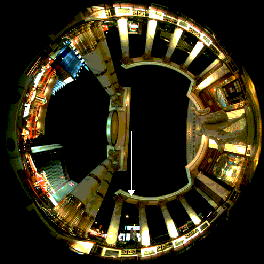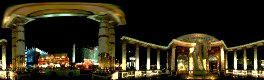Example 4: Format: Angle Horizontal Center = 0 Deg Arguments: -v 90,-20 -h 270 -f angle Diagram Rotate So That Center DirectionIs At Bottom For Easier ComparisonExample 5: Format: Tilt (Perspective) Horizontal Center = 180 Deg Persp. FOV = ifov/2 = 110 Deg Arguments: -v 90,-20 -h 180 -f tilt -p 110 Diagram Rotate So That Center DirectionIs At Bottom For Easier ComparisonExample 6: Format: Level (Perspective) Horizontal Center = 180 Deg Persp. FOV = ifov/2 = 110 Deg Arguments: -v 90,-20 -h 180 -f level -p 110 Diagram Rotate So That Center DirectionIs At Bottom For Easier Comparison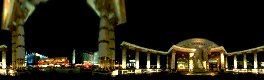Example 7: Format: Level (Perspective) Horizontal Center = 180 Deg Persp. FOV = ifov/2 = 110 Deg Output Extended 150% Vertically Arguments: -v 90,-20 -h 180 -f level -p 110 -e 150 Diagram Rotate So That Center DirectionIs At Bottom For Easier ComparisonExample 8: Format: Angle Horizontal Center = 180 Deg Persp. FOV = ifov/2 = 110 Deg Arguments: -v 90,-20 -h 180 -f angle -w 500 Diagram Rotate So That Center DirectionIs At Bottom For Easier Comparison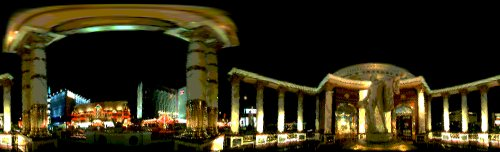Example 9: Format: Tilt (Perspective) Horizontal Center = 180 Deg Persp. FOV = ifov/2 = 110 Deg Arguments: -v 90,-20 -h 180 -f tilt -w 500 Diagram Rotate So That Center DirectionIs At Bottom For Easier ComparisonSimilar Results Using Multiple IM "Convert" Steps Including The New IM -distort depolar Note: all the steps below could be put into one command line which would prevent excess resampling/blurring Note: the roll step can be avoided as it can be folded into the -distort depolar by providing the correct parameters Hyperwide (Panoramic) Fisheye Image Panoramic Vertical View Range: -62.5 deg to 52.5 deg = 115 deg Fisheye Field Of View: 2*(52.5 - (-90)) = 285 deg Nadir At Center http://www.0-360.com/index.asp OriginalDiagram Of LookDirection For PanoramaStep 1: Convert Fisheye To Panorama Arguments: -depolar 0Step 2: Roll to put look direction 211.5 deg at center and Flip (as nadir is at center of fisheye) rollx=width*(211.5-180)/360=37.185 Arguments: -roll +37.185+0 -flip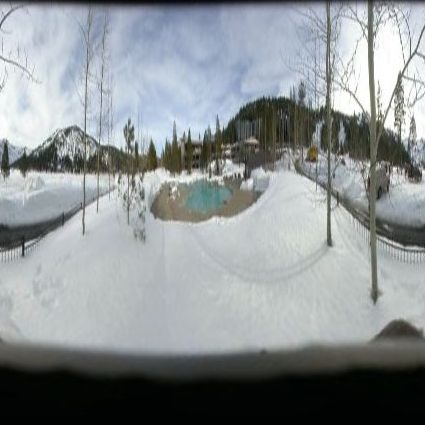Step 3: Resize for desired width of 600 and to correct angular aspect ratio aspect=vrange/180=142.5/360=0.395833 wfactor=100*desiredwidth/imagewidth=100*600/425=141.176 hfactor=aspect*wfactor=0.385833*141.176=55.8821 Arguments: -resize 141.176x55.8821%Step 4: Correct to proper tilted planar perspective compute inverse of perspective focal length from vrange vrange=(vrad-vcen)=(52.5 - (-90)) = 142.5 deg pfinv=(2*tan(vrange*pi/360))/height=0.02486 Arguments: convert testimage2_depolar0_flip_roll_resize.jpg \ -monitor -fx "yd=j-h/2; ys=(180*h/(pi*\$vrange))*atan(\$pfinv*yd)+h/2; u.p{i,ys}" \ testimage2_depolar0_flip_roll_resize_persp.jpgWhat the script does is as follows: For a linear circular fisheye distortion, it applies the following equations: perspective: r=f*tan(phi); f=(N/2)/tan((fov/2)*(pi/180)) fisheye: r=f*phi; f=(N/2)/((fov/2)*(pi/180)) where N=smaller dimension This is equivalent to the following IM commands for the case of a linear circular 180 deg fisheye image of size 362x362 extending from nadir at the center to horizontal at its radius=181 and a tilt perspective fov = 90 (ifov/2) looking such that the top of the fisheye (0 deg) will be in the center of the output. dim=362" yc=181" yd="yd=j-\$yc;" pfov=90 pfoc=`convert xc: -format "%[fx:\$dim/(2*tan(\$pfov*pi/360))]" info:` phicenter=-90 phiradius=0 ifov=`convert xc: -format "%[fx:2*abs(\$phiradius-\$phicenter)]" info:` aspect=`convert xc: -format "%[fx:360/\$ifov/]" info:` height=`convert xc: -format "%[fx:\$aspect*\$dim]" info:` phioffset=`convert xc: -format "%[fx:(pi/180)*abs((\$phiradius-\$phicenter))/2]" info:` phi="phi=atan2(yd,\$pfoc)+\$phioffset;" rr="rr=\$aspect*\$ifoc*phi;" theta0=`convert xc: -format "%[fx:(\$thetacenter-(\$thetarange/2)-90)*pi/180]" info:` theta1=`convert xc: -format "%[fx:(\$thetarange)*pi/180]" info:` theta="theta=\$theta1*(i/(w-1))+\$theta0;" xs="xs=rr*cos(theta)+\$xc;" ys="ys=rr*sin(theta)+\$yc;" convert \( -size \${dim}x\${height} xc: \) \$infile \ -virtual-pixel \$vpmethod -background \$bgcolor -monitor \ -fx "\$yd \$phi \$rr \$theta \$xs \$ys v.p{xs,ys}" \ \$outfile